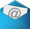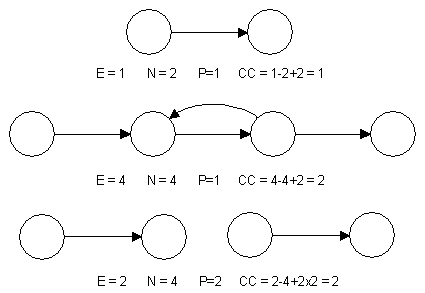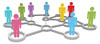Definition Email this page to a friend# (Alias: McCabe number)

Fools ignore complexity. Pragmatists suffer it. Some can avoid it. Geniuses remove it.
- Alan Perlis, American Scientist

McCabe's cyclomatic complexity is a software quality metric that quantifies the complexity of a software program. Complexity is inferred by measuring the number of linearly independent paths through the program. The higher the number the more complex the code.

## The Significance of the McCabe Number

Measurement of McCabe's cyclomatic complexity metric ensures that developers are sensitive to the fact that programs with high McCabe numbers (e.g. > 10) are likely to be difficult to understand and therefore have a higher probability of containing defects. The cyclomatic complexity number also indicates the number of test cases that would have to be written to execute all paths in a program.

## Calculating the McCabe Number

Cyclomatic complexity is derived from the control flow graph of a program as follows:

Cyclomatic complexity (CC) = E - N + 2P
Where:
P = number of disconnected parts of the flow graph (e.g. a calling program and a subroutine)
E = number of edges (transfers of control)
N = number of nodes (sequential group of statements containing only one transfer of control)

# Examples of McCabe Number Calculations8 member ratings

✭ ✭ ✭ ✭ ✭

# roblox robux

## By anonymous » Thu 27-Aug-2020, 02:46, My rating: ✭ ✭ ✭ ✭ ✩

This is so useful now to get the way how to get free robux online and this website is such useful for free robux and other resources here.

8 Comments  • Page 1 of 8 •         1   2   3   4   5  …8 » Next- Rate this definition.
- Did it help?
- Suggest improvements.